# 置顶用鼠标编辑的多边形裁剪！ gizmo 插件入门 ！ Cocos Creator！精选热门

【本文使用图文素材转载自微信公众号： 白玉无冰，作者 白玉无冰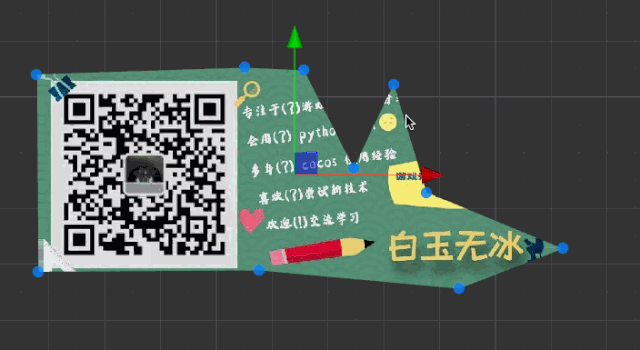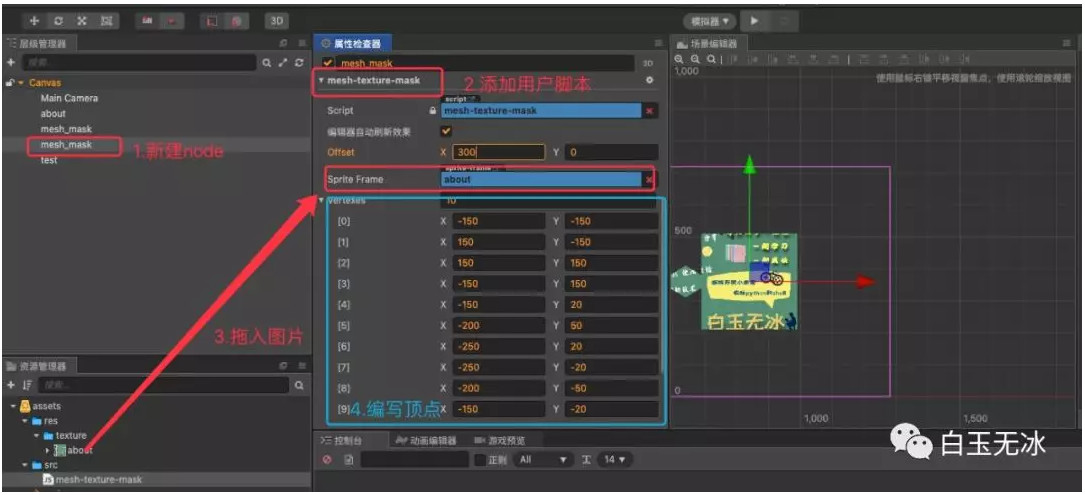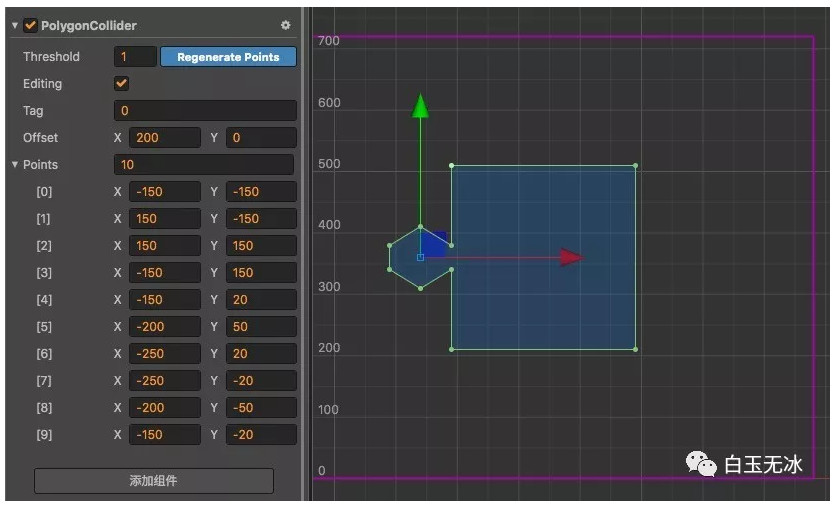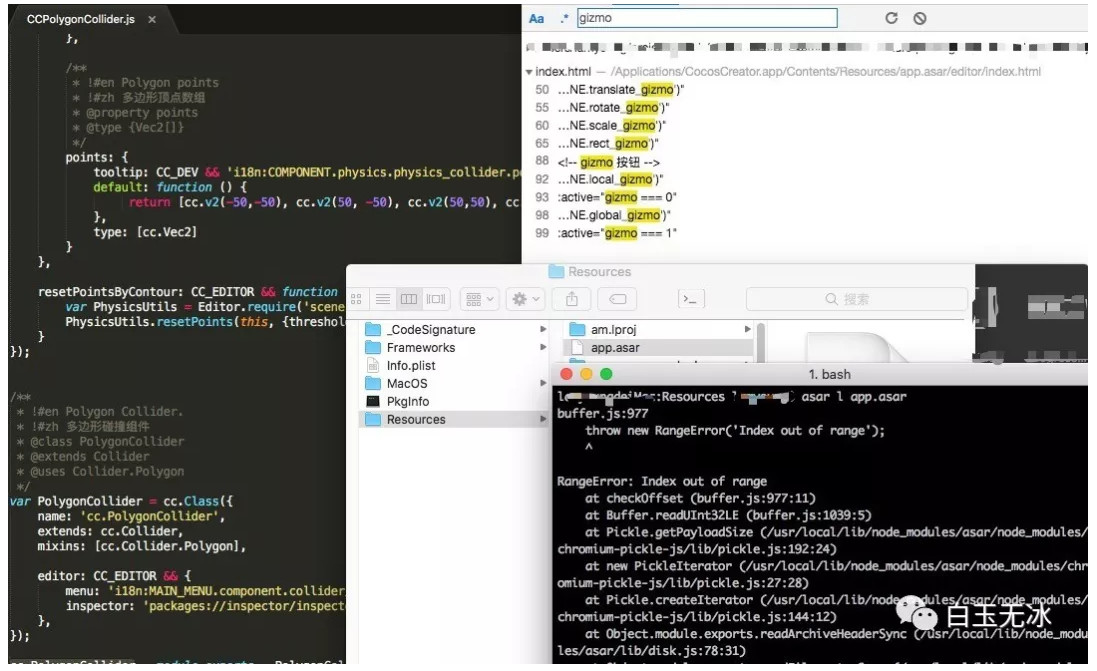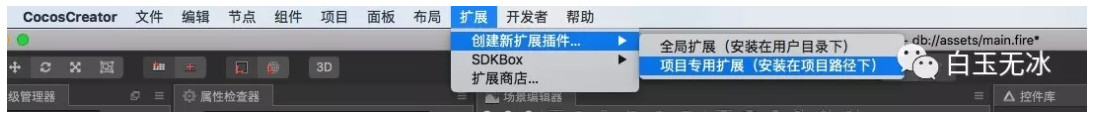```"gizmos": {
}
```

```this._tool.plot = (points, position) => {
// 移动到节点位置
this._tool.move(position.x, position.y);
// 清除原来的点
// 画圆点
points.map((v, i) => {
// this._view.scale 编辑器缩放系数
v = Editor.GizmosUtils.snapPixelWihVec2(v.mul(this._view.scale));
let circle = circles[i];
if (!circle) {
circles[i] = circle = this._tool.circle()
// 设置 fill 样式
.fill({ color: 'rgba(0,128,255,0.8)' })
// 设置点击区域，这里设置的是根据 fill 模式点击
.style('pointer-events', 'fill')
// 设置鼠标样式
.style('cursor', 'move')
// 注册点击事件
this.registerMoveSvg(circle, i, { cursor: 'pointer' });
}
})
};
```

```{
update: (dx, dy, event, i) => {
// 获取 gizmo 依附的组件
let target = this.target;
if (!start_vertex) {
start_vertex = target.vertexes[i].clone();
}
target.vertexes[i].x = start_vertex.x + dx / this._view.scale;
target.vertexes[i].y = start_vertex.y + dy / this._view.scale;
target.vertexes = target.vertexes;
}
}
```

【本文使用图文素材转载自微信公众号： 白玉无冰，作者 白玉无冰